# Summation of Fourier series

(diff) ← Older revision | Latest revision (diff) | Newer revision → (diff)

The construction of averages of Fourier series using summation methods. The best developed theory of the summation of Fourier series is that which uses the trigonometric system. In this case, for functions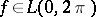with Fourier series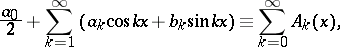the properties of the averages corresponding to the summation method are studied. For example, for the Abel–Poisson summation method, the averages are harmonic functions in the unit disc: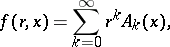while for the summation method of arithmetical averages (cf. Arithmetical averages, summation method of), they are the Fejér sumsApart from these, the most important in the theory of one-dimensional trigonometric series are the Cesàro summation methods, the Riesz summation method, the Riemann summation method, the Bernstein–Rogosinski summation method, and the de la Vallée-Poussin summation method. Summation methods that are generated by a more-or-less arbitrary sequence of-multipliers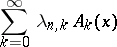have also been studied.

The summation of Fourier series is used in the following problems.

## Representations of functions using Fourier series.

For example, the Abel–Poisson averages, when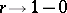, and the Fejér sums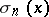, when, converge to the functionat its points of continuity; they converge moreover uniformly ifis continuous at all points; for every function, these averages converge to the function in the metric ofand almost certainly. The partial sums of a Fourier series do not possess these properties.

## Construction of polynomials with good approximation properties.

The Jackson inequality was established with the help of the summation of Fourier series. In order to solve this problem, as well as using known summation methods, new methods have been proposed, such as the Jackson singular integral and the de la Vallée-Poussin sums (cf. de la Vallée-Poussin sum).

Many properties of functions can be characterized in terms of averages of Fourier series. For example, a functionis essentially bounded if and only if there exists a constantsuch thatfor alland.

The summation of Fourier series plays an essential part in the theory of multiple trigonometric series. Thus, instead of spherical partial sums, their Riesz means of sufficiently high order are often used.

Summation of Fourier series is also examined with respect to other orthonormal systems of functions — both concrete systems and classes of systems, for example, orthogonal polynomials, as well as arbitrary orthonormal systems.

How to Cite This Entry:
Summation of Fourier series. Encyclopedia of Mathematics. URL: http://encyclopediaofmath.org/index.php?title=Summation_of_Fourier_series&oldid=19045
This article was adapted from an original article by S.A. Telyakovskii (originator), which appeared in Encyclopedia of Mathematics - ISBN 1402006098. See original article